# 1.需求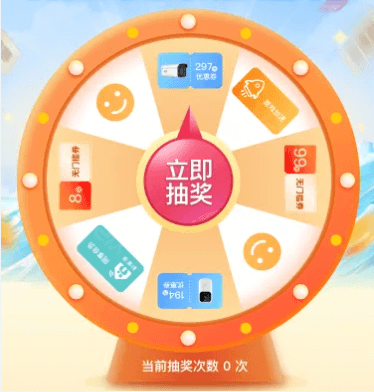# 3.实现过程

## 3.1背景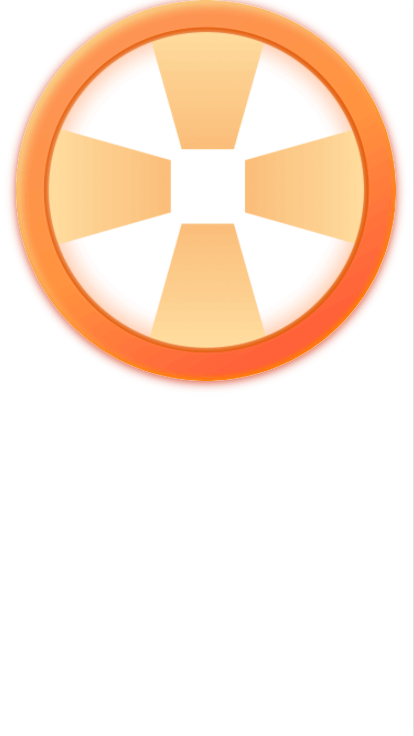## 3.2转盘跑马灯

```<!-- 转盘周围跑马灯 -->
<div class="lightWrap">
<div
v-for="i in 20"
:key="i"
:style="setLightRotate(i)"
class="lightItem">
<img
v-if="i % 2 == 0"
:class="{active: !lightChange}"
:src="lightChange ? lightGrey : lightActive"
alt=""/>
<img
v-else
:class="{active: lightChange}"
:src="!lightChange ? lightGrey : lightActive"
alt=""/>
</div>
</div>```

```//20个灯泡设置倾斜
setLightRotate(index) {
let lightRotate = (360 / 20) * index
return {
transform: ‘rotate(‘ + lightRotate + ‘deg)‘
}
}```

```//浏览器播放跑马灯动画
setTimeLine() {
this.lightCount += 1
// 浏览器渲染频率 60帧/s 约等于 16.66ms 一次，取20的倍数，就是约300ms切换跑马灯一次
if ((this.lightCount % 20) == 0) {
this.lightChange = !this.lightChange
}
requestAnimationFrame(this.setTimeLine)
}```

lightChange变量还切换了当前灯泡的样式，点亮后还会设置灯泡变大一点。css如下：

```.lightWrap {
width: \$turntableWrap_size;
height: \$turntableWrap_size;
position: absolute;
left: 50%;
top: 50%;
margin-left: calc(#{\$turntableWrap_size} / -2);
margin-top: calc(#{\$turntableWrap_size} / -2);
.lightItem {
width: 22px;
height: calc(#{\$turntableWrap_size} / 2);
position: absolute;
left: 50%;
top: 0%;
transform-origin: 0 calc(#{\$turntableWrap_size} / 2);

img {
width: 22px;
height: 22px;
position: absolute;
top: 10px;
left: 0;
}
img.active {
width: 40px;
height: 40px;
position: absolute;
top: 1px;
left: -9px;
}
}
}```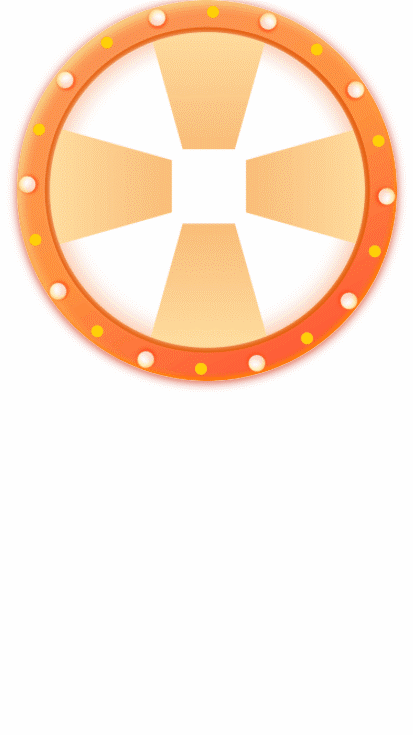## 3.3奖品图片

```<!-- 奖品图片 -->
<div class="circleMax" :class="{ani: runningLock}">
<div
v-for="(item, i) in actPrizeList"
:key="i"
:style="setRotate(i)"
class="spin">
<div :style="setSpinInner(i)" class="spinInner">
<div :style="spinCntDocObj" class="spinCntDoc">
<div class="spinImg">
<img :src="item.imgUrl" alt="" srcset="">
</div>
</div>
</div>
</div>
</div>```

actPrizeList就是奖品信息了，这个是从接口获取，里面有配置好的奖品图片连接。这里还是使用了一个方法setTotate(i)来动态设置样式，方法如下：

```/* 奖品图片倾斜 */
setRotate(index) {
let spinRotate = this.jiaodu * index
return {
transform: ‘rotate(‘ + spinRotate + ‘deg)‘
}
}```

setSpinInner()方法的功能类似，如下：

```setSpinInner(index) {
return {
transform: ‘rotate(-‘ + this.jiaodu + ‘deg)‘,
borderLeft: 0
}
}```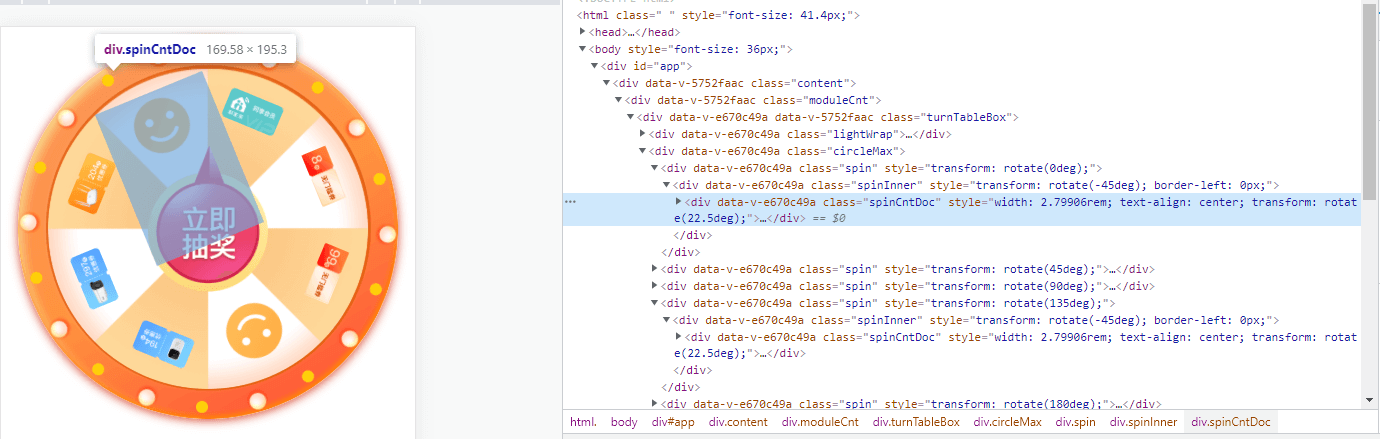```/* 图片旋转 */
setSpinCntDoc() {
let spinCntDocWidth = (Math.sin((this.jiaodu / 2) * (Math.PI / 180)) * this.tableInnerSize) / 70
this.spinCntDocObj.width = spinCntDocWidth + ‘rem‘
this.spinCntDocObj.textAlign = ‘center‘
this.spinCntDocObj.transform = ‘rotate(‘ + (this.jiaodu / 2) + ‘deg)‘
}```

## 3.4背景整体旋转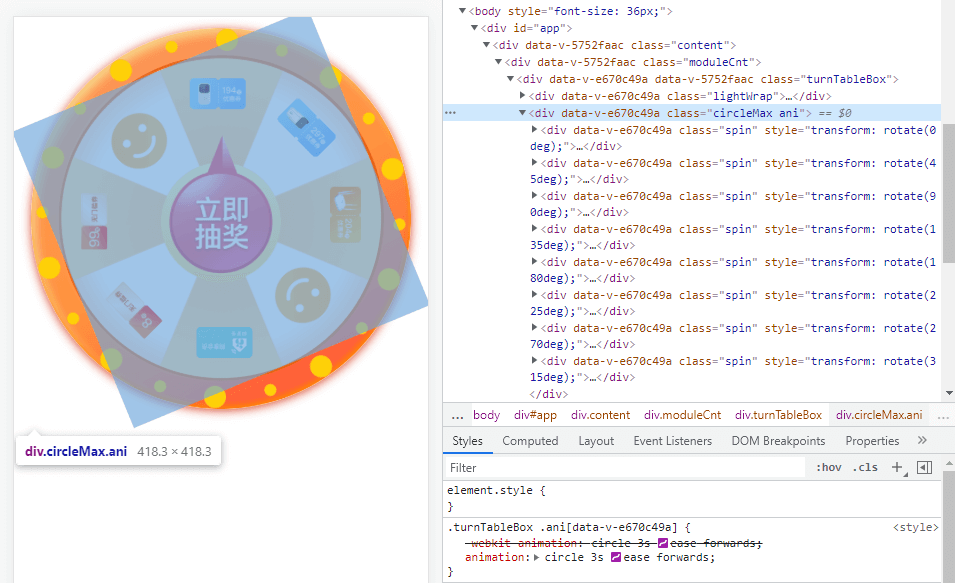css类anti中包含一个animation动画，如下：

```  .ani {
animation: circle 3s ease forwards;
}```

## 3.5抽奖按钮

```<!-- 抽奖按钮 -->
<div
class="arrowBtn"
:class="{btnShake: btnShakeShow}"
@click="startClick">
<img src="../assets/images/summer/btn-draw.png" alt=""/>
</div>```

css代码如下

```.arrowBtn {
width: 216px;
height: 260px;
text-align: center;
position: absolute;
left: 50%;
top: 50%;
margin-left: -108px;
margin-top: -152px;
img {
width: 100%;
}
}```

```.btnShake {
animation: btnShakeAni 0.5s ease-out forwards;
}
@keyframes btnShakeAni {
0% {
transform: scale(1);
}
10% {
transform: scale(1.1);
}
30% {
transform: scale(0.9);
}
50% {
transform: scale(1.1);
}
70% {
transform: scale(0.9);
}
90% {
transform: scale(1.1);
}
100% {
transform: scale(1);
}
}```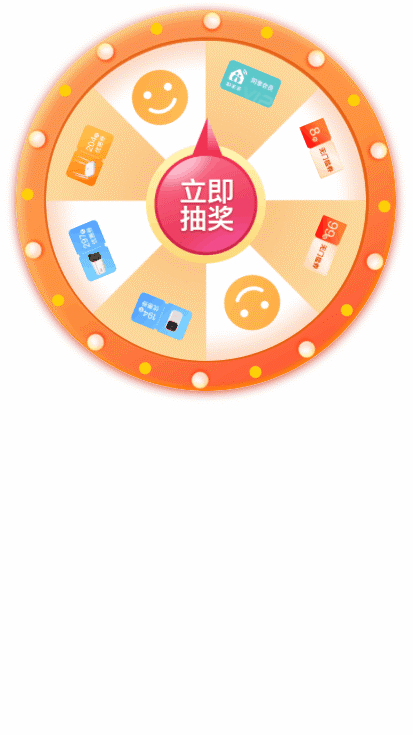## 3.6点击抽奖

```/* 点击抽奖播放动画 */
startClick() {
//大转盘旋转
this.runningLock = true
//抽奖按钮弹一下
this.btnShakeShow = true
//调接口
let data = {actCode: actCode}
coc2.drawLottery(data).then(res => {
if (res.code == 0) {
if (res.data) {
this.prizeNum = this.actPrizeList.findIndex((item, index) => item.pid == res.data.pid)
this.prizeName = res.data.prizeName
this.prizeImgSrc = res.data.litimgUrl
}
}
//动态加载动画关键帧
let targetDeg = 360 * this.defaultRunTimes + (this.spinNum - this.prizeNum) * this.jiaodu + this.jiaodu * 0.5
let runkeyframes = `@keyframes circle{ 0% {transform: rotate(0deg);} 100% {transform: rotate(\${targetDeg}deg); }`
document.getElementById(‘mystyle‘).innerHTML = runkeyframes
setTimeout(() => {
}, 3500)
})
}```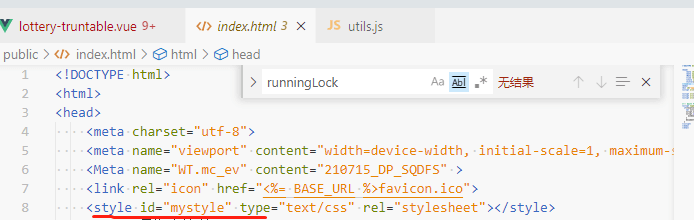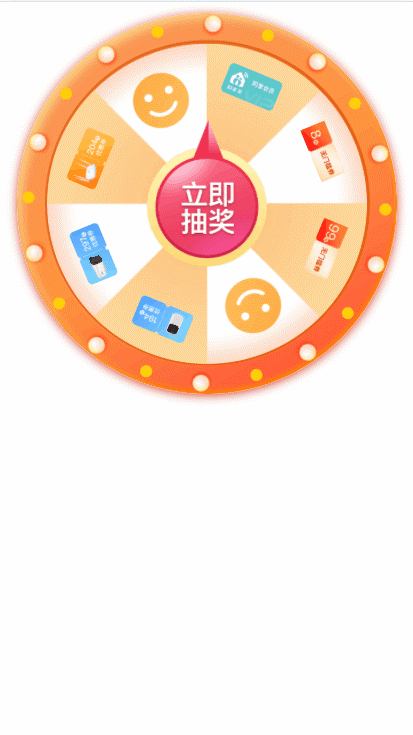## 3.7动画复原&中奖弹框

```<!-- 中奖弹框 -->
:prize-img-src="prizeImgSrc"

`EventBus.\$emit("turntableReset")`

```mounted() {
// 弹窗关闭 重置大转盘
EventBus.\$on("turntableReset", () => this.turnTableReset())
},
methods{
//转盘数据重置
turnTableReset() {
this.startLock = false
this.runningLock = false
this.btnShakeShow = false
}}```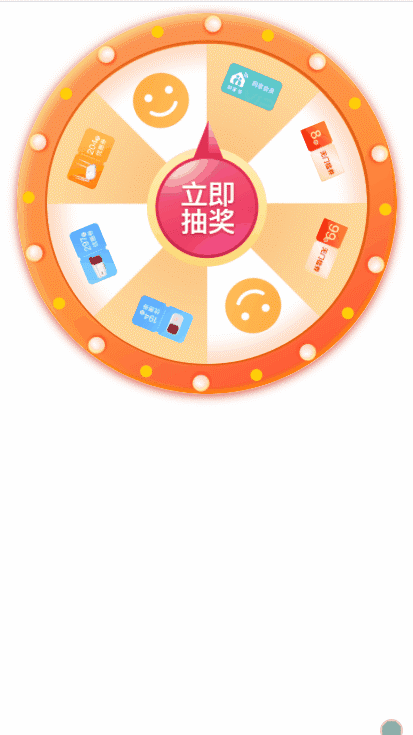# 4.总结

(0)
(0)